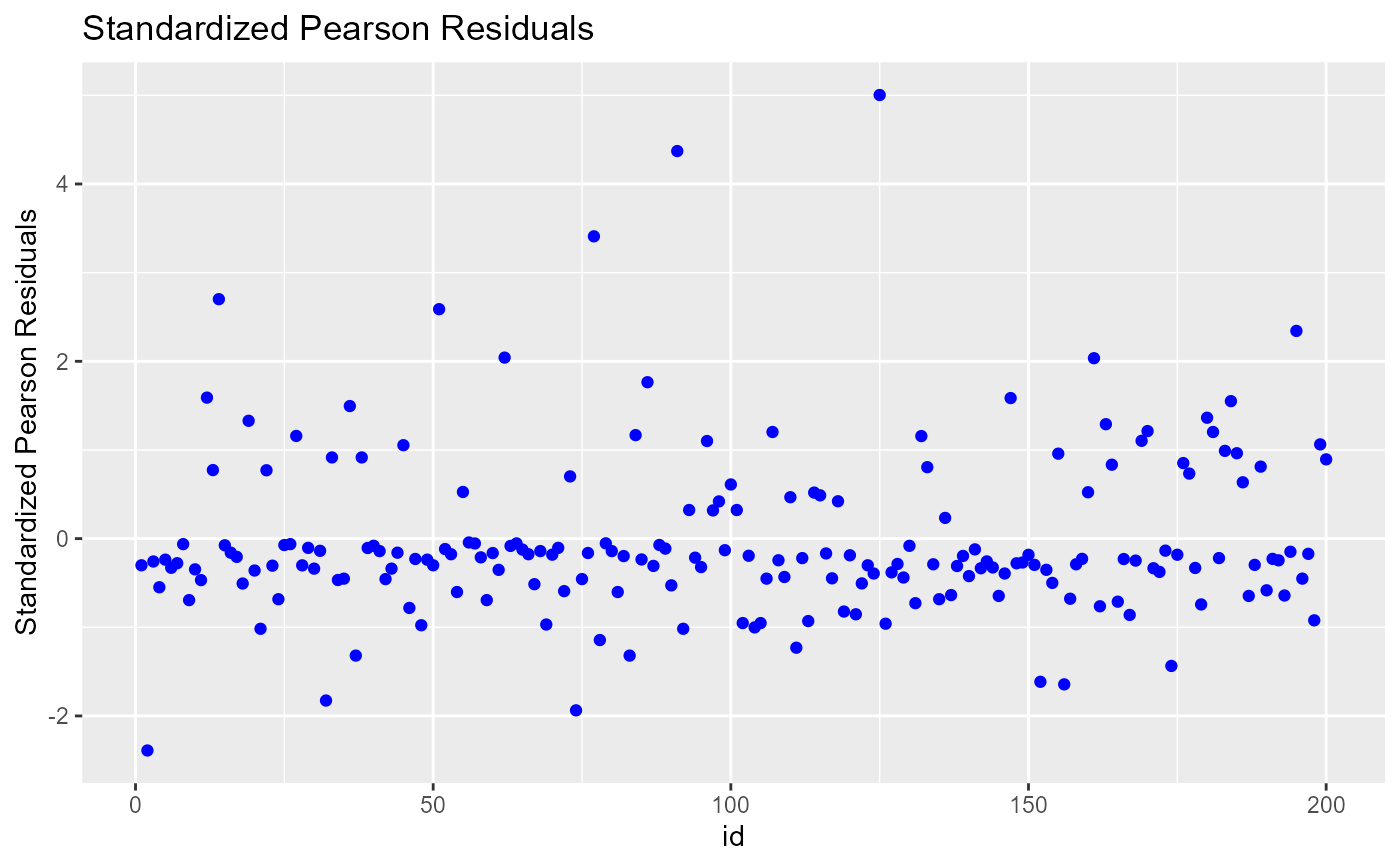Standardised pearson residuals plot.

blr_plot_pearson_residual(
model,
point_color = "blue",
title = "Standardized Pearson Residuals",
xaxis_title = "id",
yaxis_title = "Standardized Pearson Residuals"
)

## Arguments

model An object of class glm. Color of the points. Title of the plot. X axis label. Y axis label.

## Examples

model <- glm(honcomp ~ female + read + science, data = hsb2,
family = binomial(link = 'logit'))

blr_plot_pearson_residual(model)